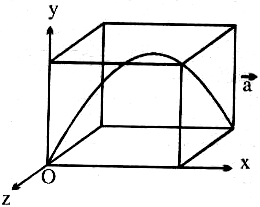# The answer to each of the questions is a single digit integer ranging from 0 to 9.A cubical box dimensional L = 5/4 metre starts moving with an acceleration a ? = 0.5 m/s2 i ^ from the state of rest. At the same time, a stone is thrown from the origin with velocity v ?=v_1 i ^+v_2 j ^-v_3 k ^ with respect to earth. Acceleration due to gravity g ? = 10 m/s2 (-j ^ ). The stone just touches the roof of box and finally falls at the diagonally opposite point then find the value of v_1/v_3 -1/v_2 .9 years ago

1

H = (u_y^2)/g

uy = 5 m/s    => v2 = 5 m/s

T = (2u sinx)/g=(2u_y)/g = 1 sec

S = u × t -1/2 a × t2 (as a ? in x direction)

5/4 = ux × 1 -1/2 × (0.5) × 1

ux = 3/2 m/sec,    => v1 = 3/2 m/s

Along z-axis, 5/4 = v3 × 1 => v3 = 5/4 m/sec.

v_1/v_3 -1/v_2 =(3/2×4/5)-1/5 = 1